# Diagram solving equations, change of variables

## Solving equations using equations and effect

1. Conversion, guaranteeing the preservation of the right of equality
2. Checking the roots by substitution into the original equation

## Solving equations by using equivalent transformations

1. Note IDS equal for both forward and reverse transformations
2. Save on DHS equal for both forward and reverse transformations

## The solution of equations. The change of variables

If in the equation variable occurs in the same form, whether the corresponding expression with a variable to mark one letter (a new variable).

### Example 1.

Rozwarte equation: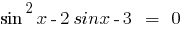Rozwiazania.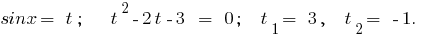1. Ifthe equation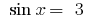has no roots because2. If you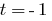have, then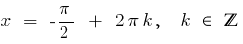Answer: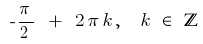### Example 2.

Rozwarte equation:Rozwiazania. Because under the root sign pair forms can stand alone newmn expression, then the region of acceptable values (IDS) of the given equation is given by a system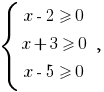rozvyazka which is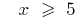The product is equal to zero if and only if one of the multipliers equal to zero, and all other multipliers exist: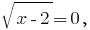when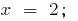when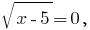when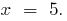The value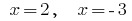is not included in the odz of the given equation, therefore, they are not the roots of the given equation.

The value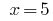included in the DHS, so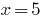is a root of the given equation.

Answer:Tags:
Chapter:
Versions in other languages:
Share with friends: